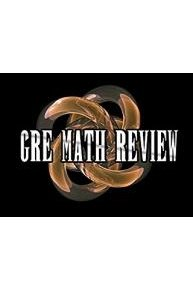# Watch GRE Math Review

The following series of videos will familiarize you with the mathematical skills and concepts that are important to know, for the purpose of solving problems on the quantitative reasoning section of the GRE revised General Test. GRE Math Review is a series that is currently running and has 1 seasons (9 episodes). The series first aired on .

GRE Math Review is available for streaming on the Math Fortress website, both individual episodes and full seasons. You can also watch GRE Math Review on demand at Amazon Prime online.

Math Fortress
1 Season, 9 Episodes
iWatch Episodes

## GRE Math Review Full Episode Guide

• This video is a review of basic arithmetic for the purpose of solving problems on the quantitative reasoning section of the GRE revised General Test. This video covers the basics of fractions. Topics covered: Comparing Fractions, Comparing more then two Fractions, fractions with irrational Numbers, combination of operations with fractions.

• This video is a review of basic arithmetic for the purpose of solving problems on the quantitative reasoning section of the GRE revised General Test. This video covers the basics of fractions. Topic covered: division of fractions, complex fractions, and mixed numbers.

• This video is a review of basic arithmetic for the purpose of solving problems on the quantitative reasoning section of the GRE revised General Test. This video covers the basics of fractions. Topic covered: multiplication of fractions.

• This video is a review of basic arithmetic for the purpose of solving problems on the quantitative reasoning section of the GRE revised General Test. This video covers the basics of fractions. Topics covered include: addition and subtraction of fractions.

• This video is a review of basic arithmetic for the purpose of solving problems on the quantitative reasoning section of the GRE revised General Test. This video covers the basics of fractions. Topics covered include: The definition of a fraction and properties of fractions.

• This video is a review of basic arithmetic for the purpose of solving problems on the quantitative reasoning section of the GRE revised General Test. This video covers the basics of integers. Topics covered include: Even and Odd Integers, Sum of Even and Odd Integers, Product of Even and Odd Integers, Prime Numbers, Prime Factorization, and Composite Numbers.

• This video is a review of basic arithmetic for the purpose of solving problems on the quantitative reasoning section of the GRE revised General Test. This video covers the basics of integers. Topics covered include: Division, Division as a Quotient and Reminder and Divisibility of Integers.

• This video covers the basics of integers. Topics covered include: Multiplication, Multiples, Factors (Divisors), Lowest Common Multiple (LCM), and the Greatest Common Divisor (GCD) also known as the Greatest Common Factor (GCF).

• This video is a review of basic arithmetic for the purpose of solving problems on the quantitative reasoning section of the GRE revised General Test. This video covers the basics of integers. Topics covered include: Number Lines, Operations, Absolute Values, Addition and Subtraction of integers.